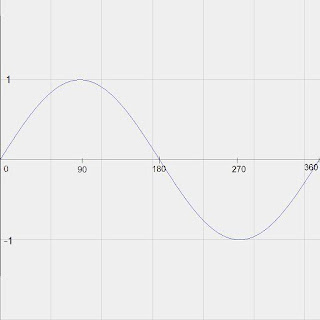# Graphing Sine and Cosine Curves

As I usually include in most of my posts, please feel free to ask questions or leave comments on any material that I post, and I will try my best to address them. I recently received a request to go over how to graph sine and cosine curves.

Sine and cosine functions are PERIODIC FUNCTIONS, meaning that the function will evaluate to a repeated number after a defined length (period). You've probably seen these graphs several times before and not realized what they were. Sine and cosine graphs are virtually identical, EXCEPT that they are slightly shifted relative to one another... or they are "out of phase." This is an important distinction to make, as confusing which is which, of course, will get you the wrong answer.

The EASIEST way to remember, or to quickly plot out the graph, is to create a table of values. Let's work on the Sine curve first. The cosine one works exactly the same way. Creating this table of values will be a good review of how these trig functions work. On that note, we will evaluate for values for x that represent the angles of our special triangles, and then continue with more values at the same intervals. (I'm going to represent the square root function by "sq.rt.") If you want, you can continue to make the list longer if you wish, but I think this is sufficient to demonstrate the graph.

y = sin(x)

x |||||y
-----------
0 |||||0
30 |||||0.5
60 |||||(sq.rt.3)/2
90 ||||| 1
120||||| (sq.rt.3)/2
150||||| 0.5
180||||| 0
210||||| -0.5
240||||| -(sq.rt.3)/2
270||||| -1
300||||| -(sq.rt.3)/2
330||||| -0.5
360||||| 0

As you can probably see from looking at the list of values, the numbers seem to have a repeating pattern. If you continue to add values, they will begin to repeat themselves again. Essentially, we have gone in a full circle (360 degrees), and so that is why they repeat.

So, taking these values that we have and plotting them on a graph, we can see what a sine curve looks like:The cosine curve will look very similar, and you can deduce it exactly the same way. As I said before, they look the same but are out of phase. The important difference to remember is that the sine curve passes through (0,0), and the cosine curve passes through (0,1), but the 'periodicity' is the same... that is, they repeat at the same regular intervals. You can extend the curve into the negative x direction, or further in the positive direction.

I hope that explains what the original poster was asking me to go over. These functions, like any other function, can also be modified by adding coefficients etc, in the same way as other functions that I've reviewed before. I will explain these concepts in a future post. Or for fun, add a coefficient to the front of the sine function (eg. A*sin(x)), or to the x value itself (sin(2x)) and create tables of values and compare the resulting graphs!